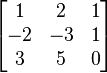## N*N Rank of Matrix Calculator

 Select Matrix dimension: 2345678910 X
 Matrix rank is

The rank of a matrix A is The maximum number of linearly independent rows in a matrix A (the row rank) or the maximum number of linarly independent columns in A (the column rank).

What is not so obvious, However For every matrix, the column rank is equal to the row rank. So Get one rank of matrix we will get the rank of matrix. The rank is one of the fundamental pieces of data associated with a matrix.

### ExamplesThe rank is commonly denoted rank(A) or rk(A); Because all column of the matrix if linearly independent, so rank(A) = 3.

Thinkcalculator.com provides you helpful and handy calculator resources.